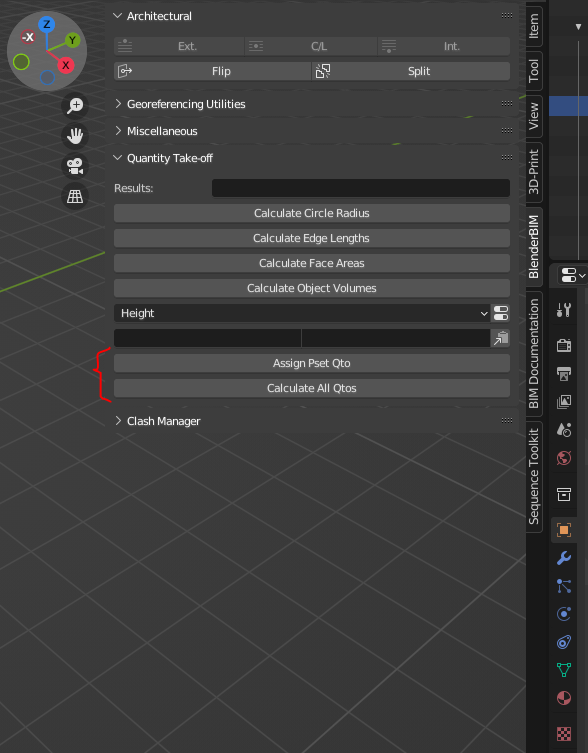# Bulk Guess Quantities for IfcSpaces

Hi,
I'm triing to write a script to populate Quantities for all IfcSpaces of an IFC model. I've seen that as a user I can do it through ObjectProperties/...IfcObjectQuantitySets and editing and pressing the 'Guess Quantity' button. Which method should I call when scripting to achive this.
As I can see in the info panel I should get the object, enable pset editing and then guess the quantity of a property. Is this a correct aproach?
Btw theres is API Documentation (or something similar) for IfcOpenshel Python and Blender BIM ?
Any clue would be apreciated!!

• Hi @vbertran , if you want i created, in a new branch, two buttons in qto n-panel that basically apply guess quantities for every selected object (https://github.com/maxfb87/IfcOpenShell)
Maybe the code can be useful for your purpose.
Btw, guess quantity is only an approximation (sometimes wrong) to calculate quantities and @vukas is trying to improve this aspect.

• Hi @Massimo ! Thank you very much for your response and the link. I'll take a look.
I have also detected that guess quantity does not calculate allways right. As far as I know and in the case of IfcSpace WallAreas and CeilingAreas are not well computated.

• Hey @vbertran , if you want to try, in the new release of BBim (yesterday) there are 2 new buttons that can help you.Also, when @vpajic will finish his work, there will be others calculations methods :-)

• Great @Massimo! Thanks. As soon as I have the moment I'll check it. I'll late you know.

• edited October 2022

Hi @Massimo, @vpajic.
I've been testint the 'Assinng Pset Qto' and 'Calculate All Qtos' buttons. First of all I must say that's a great feature and it's been working quiet well. Congratulations!
I've been testing assigning quantities just to IfcSpaces of 3 diferent models ut to 550 spaces. With the largest model it took a while (about 2 minuts to complete the operation). I didn't try to assing quantites to a whole model.
I've also been checking the output results with BIM Vision IFC viewer and it worked quiet well.
Regarding to IfcSpaces I've noticed that 'Net/GrossCeilingArea' and 'Net/GrossWallArea' is not assigned correctly it seems that it calculates the entire object's area.
For future developments maybe it would be nice for the user to decide which classes and which quantities to calculate (and not all classes and all quantities).
Anyway. It's great!

• @vbertran thanks, i'm very glad that it helped you :-)
I'm trying to improve the speed, but i don't get it. The strange thing is that if i run the same code in the script instead of as blender operator, it's faster. Btw i'm keep trying.
About the calculations, indeed they should be better calculated.
Thanks also for suggestions, i keep the note :-)

• @vbertran - Yes, the values calculated can often be wrong, since they are in fact "guesses" of what the quantity should be. If you want more control over the quantity, I added additional functions to QtoCalculator: https://github.com/IfcOpenShell/IfcOpenShell/blob/v0.7.0/src/blenderbim/blenderbim/bim/module/pset/qto_calculator.py

I haven't had time to fully document the features, but it's on my todo list. Here's a snippet of what's possible:

``````        #qto = QtoCalculator()
#o = bpy.context.active_object
#sel = bpy.context.selected_objects
#
#nl = '\n'
# print(
#     f"get_linear_length: {qto.get_linear_length(o)}{nl}{nl}"
#     f"get_width: {qto.get_width(o)}{nl}{nl}"
#     f"get_height: {qto.get_height(o)}{nl}{nl}"
#     f"get_perimeter: {qto.get_perimeter(o)}{nl}{nl}"
#     f"get_lowest_polygons: {qto.get_lowest_polygons(o)}{nl}{nl}"
#     f"get_highest_polygons: {qto.get_highest_polygons(o)}{nl}{nl}"
#     f"get_net_footprint_area: {qto.get_net_footprint_area(o)}{nl}{nl}"
#     f"get_net_roofprint_area: {qto.get_net_roofprint_area(o)}{nl}{nl}"
#     f"get_side_area: {qto.get_side_area(o)}{nl}{nl}"
#     f"get_total_surface_area: {qto.get_total_surface_area(o)}{nl}{nl}"
#     f"get_volume: {qto.get_volume(o)}{nl}{nl}"
#     f"get_opening_area(o, angle_z1=45, angle_z2=135, min_area=0, ignore_recesses=False): {qto.get_opening_area(o, angle_z1=45, angle_z2=135, min_area=0, ignore_recesses=False)}{nl}{nl}"
#     f"get_lateral_area(o, subtract_openings=True, exclude_end_areas=False, exclude_side_areas=False, angle_z1=45, angle_z2=135): {qto.get_lateral_area(o, subtract_openings=True, exclude_end_areas=False, exclude_side_areas=False, angle_z1=45, angle_z2=135)}{nl}{nl}"
#     f"get_gross_top_area: {qto.get_gross_top_area(o, angle=45)}{nl}{nl}"
#     f"get_net_top_area(o, angle=45, ignore_internal=True): {qto.get_net_top_area(o, angle=45, ignore_internal=True)}{nl}{nl}"
#     f"get_projected_area(o, projection_axis='z', is_gross=True): {qto.get_projected_area(o, projection_axis='z', is_gross=True)}{nl}{nl}"
#     f"get_OBB_object: {qto.get_OBB_object(o)}{nl}{nl}"
#     f"get_AABB_object: {qto.get_AABB_object(o)}{nl}{nl}"
#     f"get_bisected_obj(o, plane_co_pos=(0,0,1), plane_no_pos=(0,0,1), plane_co_neg=(0,0,1), plane_no_neg=(0,0,1)): {qto.get_bisected_obj(o, plane_co_pos=(0,0,1), plane_no_pos=(0,0,1), plane_co_neg=(0,0,1), plane_no_neg=(0,0,1))}{nl}{nl}"
#     f"get_total_contact_area(o, class_filter=['IfcWall', 'IfcSlab']): {qto.get_total_contact_area(o, class_filter=['IfcWall', 'IfcSlab'])}{nl}{nl}"
#     f"get_touching_objects(o, ['IfcElement']): {qto.get_touching_objects(o, ['IfcElement'])}{nl}{nl}"
#     #f"get_contact_area: {qto.get_contact_area(o)}{nl}{nl}"
#     )`
``````
• Great @vpajic, thanks for sharing.
From what I can understand reading the code of QtoCalculator Class, depending on the name of the Property it calls one method or another. Am I right?
So in the case of 'Net/Gross Wall Area' it should call 'get_side_area' (or 'get_lareal_area'?) method but it's calling 'get_total_surface_area' instead. And something similar it's happening with the 'Net/GrossCeilingArea'